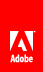# y

Determines the y coordinate of a given form design object relative to its parent object.

#### Syntax

`Reference_Syntax.y( OBJECT param1 [, STRING param2 [, INTEGER param3 ] ] )`

#### Parameters

 param1 The fully qualified reference syntax expression of one of the following container XML Form Object Model objects: area , contentArea , draw , field , pageArea , subform . param2 (Optional) A string representing the unit type of the return value. If left blank, the default unit type is points. param3 (Optional) An integer representing a zero-based index value indicating the content area in which you want to obtain the object’s y coordinate. If left blank, the default value is 0 . This parameter allows you to calculate the y coordinate of an object that is distributed across multiple content areas, such as pages. For example, if you want to find the absolute y positioning of a subform object that spans multiple content areas, you would use this parameter to enumerate the y coordinate of the subform in each of the content areas and add the totals together. If the object for which you want to calculate a y coordinate is nested within several layers of parent objects, you must factor in the y coordinate of each parent object when computing the actual y coordinate of the object.

#### Returns

The y coordinate of the form design object as a double.

Model

Object

XFA 2.1

#### JavaScript

```// Returns the y coordinate of a single instance of TextField1, relative to
// its parent object.
xfa.layout.y(TextField1,"in");

// Calculates the y coordinate of Subform1 across two content areas and
// displays the total in a message box.
var iY = xfa.layout.y(Subform1,"in",0) + xfa.layout.y(Subform1,"in",1);
xfa.host.messageBox(iY);```

#### FormCalc

```// Returns the y coordinate of a single instance of TextField1, relative to
// its parent object.
xfa.layout.y(TextField1,"in")

// Calculates the y coordinate of Subform1 across two content areas and
// displays the total in a message box.
var iY = xfa.layout.y(Subform1,"in",0) + xfa.layout.y(Subform1,"in",1)
xfa.host.messageBox(iY)```// Ethnio survey code removed